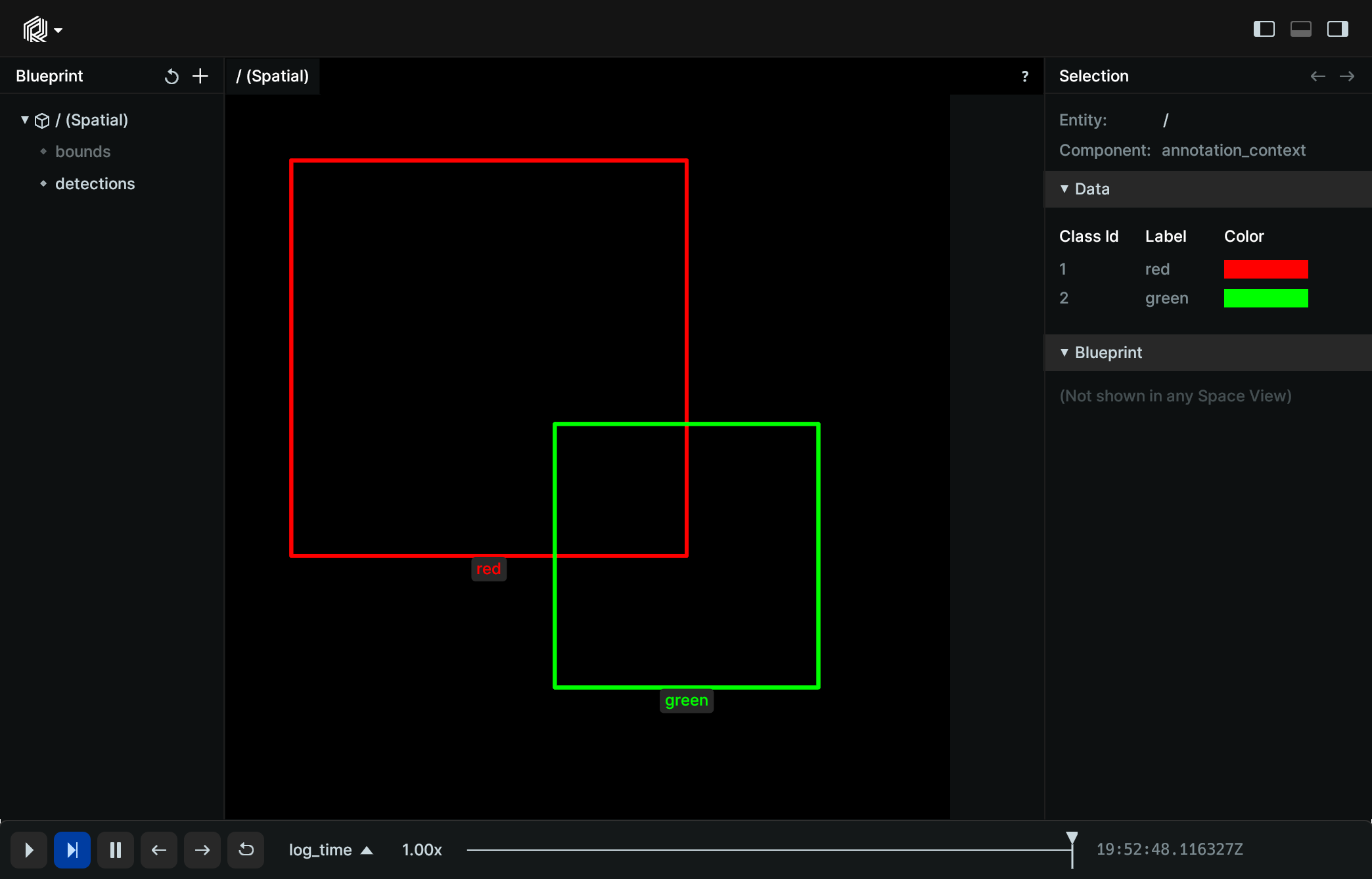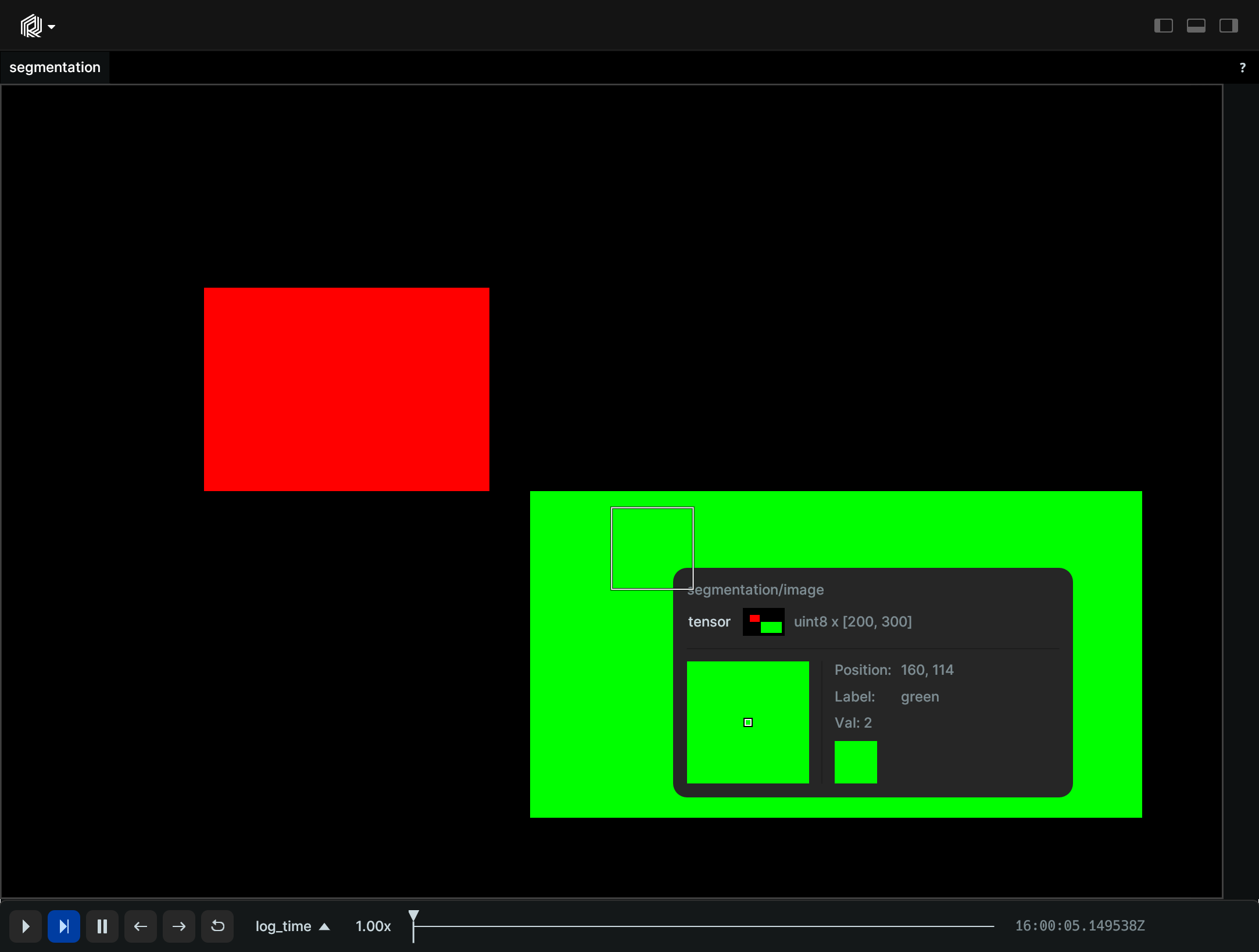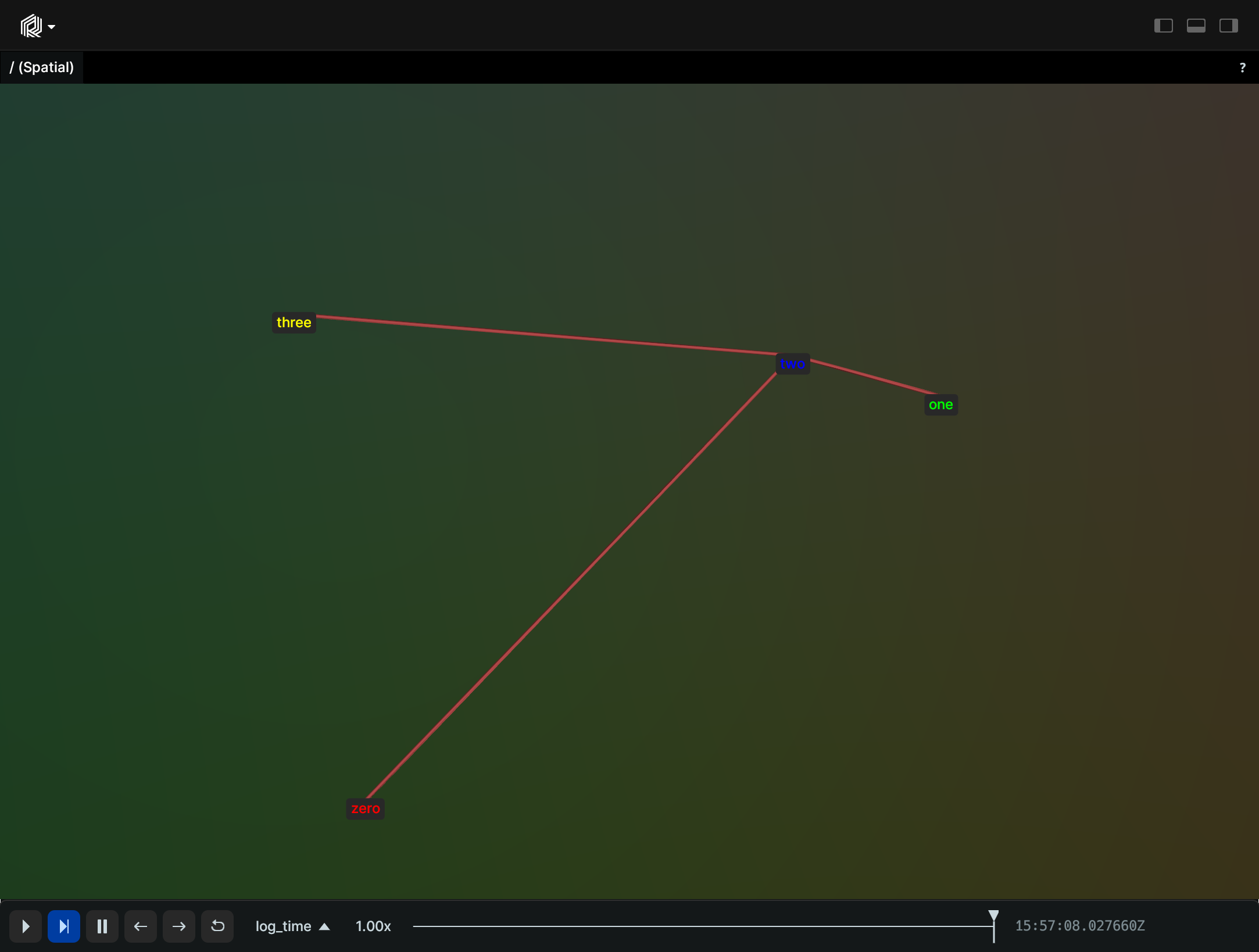# AnnotationContext

The `AnnotationContext` provides additional information on how to display entities.

Entities can use `ClassId`s and `KeypointId`s to provide annotations, and the labels and colors will be looked up in the appropriate `AnnotationContext`. We use the first annotation context we find in the path-hierarchy when searching up through the ancestors of a given entity path.

## Components

Required: `AnnotationContext`

## Examples

### Rectangles

``````import rerun as rr

rr.init("rerun_example_annotation_context_rects", spawn=True)

# Log an annotation context to assign a label and color to each class
rr.log("/", rr.AnnotationContext([(1, "red", (255, 0, 0)), (2, "green", (0, 255, 0))]), timeless=True)

# Log a batch of 2 rectangles with different `class_ids`
rr.log("detections", rr.Boxes2D(mins=[[-2, -2], [0, 0]], sizes=[[3, 3], [2, 2]], class_ids=[1, 2]))

# Log an extra rect to set the view bounds
rr.log("bounds", rr.Boxes2D(half_sizes=[2.5, 2.5]))
``````### Segmentation

``````"""Log a segmentation image with annotations."""

import numpy as np
import rerun as rr

rr.init("rerun_example_annotation_context_segmentation", spawn=True)

# Create a simple segmentation image
image = np.zeros((200, 300), dtype=np.uint8)
image[50:100, 50:120] = 1
image[100:180, 130:280] = 2

# Log an annotation context to assign a label and color to each class
rr.log("segmentation", rr.AnnotationContext([(1, "red", (255, 0, 0)), (2, "green", (0, 255, 0))]), timeless=True)

rr.log("segmentation/image", rr.SegmentationImage(image))
``````### Connections

``````"""Log annotation context with connections between keypoints."""

import rerun as rr
from rerun.datatypes import ClassDescription

rr.init("rerun_example_annotation_context_connections", spawn=True)

rr.log(
"/",
rr.AnnotationContext(
[
ClassDescription(
info=0,
keypoint_annotations=[
(0, "zero", (255, 0, 0)),
(1, "one", (0, 255, 0)),
(2, "two", (0, 0, 255)),
(3, "three", (255, 255, 0)),
],
keypoint_connections=[(0, 2), (1, 2), (2, 3)],
)
]
),
timeless=True,
)

rr.log(
"points",
rr.Points3D(
[
(0, 0, 0),
(50, 0, 20),
(100, 100, 30),
(0, 50, 40),
],
class_ids=,
keypoint_ids=[0, 1, 2, 3],
),
)
``````